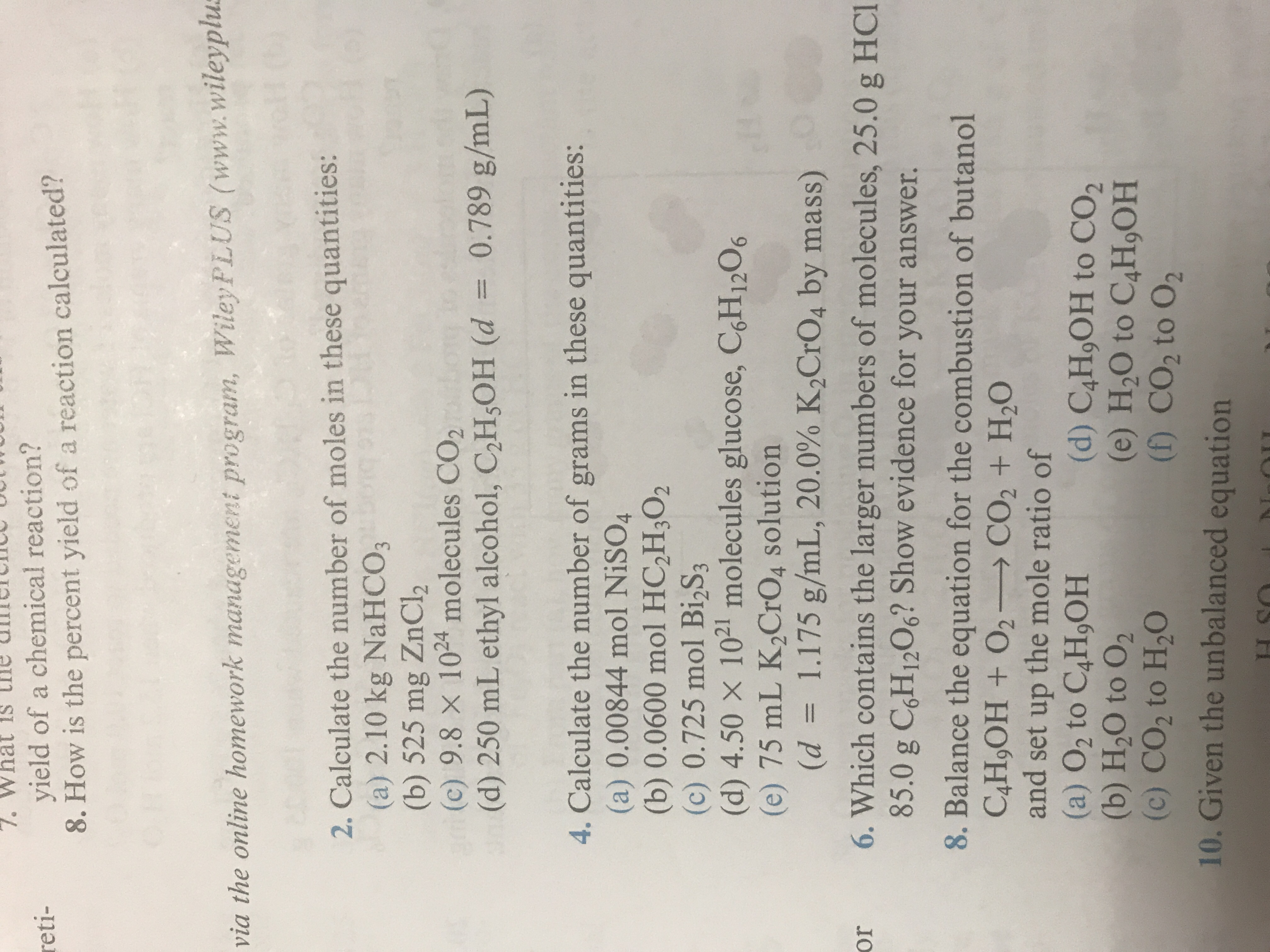# Whyield of a chemical reaction?8. How is the percent yield of a reaction calculated?retivia the online homework management program, Wiley PLUS www.wileyplu2. Calculate the number of moles in these quantities:(a) 2.10 kg NaHCO3(b) 525 mg ZnCl2(c) 9.8 × 1024 molecules CO2(d) 250 mL ethyl alcohol, C2H3OH (d = 0.789 g/mL)4. Calculate the number of grams in these quantities:(a) 0.00844 mol NiSO4(b) 0.0600 mol HC2H,O2(c) 0.725 mol Bi2S3(d) 4.50 x 1021 molecules glucose, CH1206(e) 75 mL K2CrO4 solution(d-_ 1.175 g/mL, 20.0% K2CrO4 by mass)or 6. Which contains the larger numbers of molecules, 25.0 g HCl85.0 g C,H1206? Show evidence for your answer.8. Balance the equation for the combustion of butanoland set up the mole ratio of(a) O2 to C4HOH(d) C4HoOH to CO2(b) H20 to 02(c) CO2 to H20(e) H20 to C4H,OH(f) CO2 to 0210. Given the unbalanced equation

Question

4E  how do you solve for mass when it includes percentagehelp_outlineImage TranscriptioncloseWh yield of a chemical reaction? 8. How is the percent yield of a reaction calculated? reti via the online homework management program, Wiley PLUS www.wileyplu 2. Calculate the number of moles in these quantities: (a) 2.10 kg NaHCO3 (b) 525 mg ZnCl2 (c) 9.8 × 1024 molecules CO2 (d) 250 mL ethyl alcohol, C2H3OH (d = 0.789 g/mL) 4. Calculate the number of grams in these quantities: (a) 0.00844 mol NiSO4 (b) 0.0600 mol HC2H,O2 (c) 0.725 mol Bi2S3 (d) 4.50 x 1021 molecules glucose, CH1206 (e) 75 mL K2CrO4 solution (d-_ 1.175 g/mL, 20.0% K2CrO4 by mass) or 6. Which contains the larger numbers of molecules, 25.0 g HCl 85.0 g C,H1206? Show evidence for your answer. 8. Balance the equation for the combustion of butanol and set up the mole ratio of (a) O2 to C4HOH(d) C4HoOH to CO2 (b) H20 to 02 (c) CO2 to H20 (e) H20 to C4H,OH (f) CO2 to 02 10. Given the unbalanced equation fullscreen
check_circleExpert Solution
Step 1

It is required to calculate the number of grams of K2CrO4 in K2CrO4 solution and it says that the mass of K2CrO4 is 20% of the mass of the solution. First, we need to calculate the mass of 75 mL K2CrO4 solution having a density of 1.175 g/mL, which can be done as,

Step 2

Now since the mass of K2CrO4 is 20% of the mas...

### Want to see the full answer?

See Solution

#### Want to see this answer and more?

Solutions are written by subject experts who are available 24/7. Questions are typically answered within 1 hour*

See Solution
*Response times may vary by subject and question
Tagged in

### General Chemistry